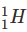Courses

Test: Hydrogen- Assertions & Reason Type Questions

7 Questions MCQ Test Chemistry Class 11 | Test: Hydrogen- Assertions & Reason Type Questions

Description
Attempt Test: Hydrogen- Assertions & Reason Type Questions | 7 questions in 14 minutes | Mock test for Class 11 preparation | Free important questions MCQ to study Chemistry Class 11 for Class 11 Exam | Download free PDF with solutions
QUESTION: 1

Direction: In the following questions, a statement of Assertion (A) followed by a statement of Reason (R) is given. Choose the correct option out of the options given below each question.Assertion (A) : Permanent hardness of water is removed by treatment with washing soda.Reason (R) : Washing soda reacts with soluble magnesium and calcium sulphate to form insoluble carbonates.

Solution: Permanent hardness of water can be removed by using washing soda. Washing soda reacts with soluble calcium and magnesium chlorides and sulphates in hard water to form insoluble carbonates.
QUESTION: 2

Direction: In the following questions, a statement of Assertion (A) followed by a statement of Reason (R) is given. Choose the correct option out of the options given below each question.Assertion: The mass number of hydrogen is equal to its atomic numberReason: H− atom has no neutrons.

Solution: For a chemical element the number of protons found in its nucleus is known as its atomic number. Atomic number is unique for each and every element so it is considered as the basis of the periodic table formation. Earlier mass numbers were considered but due to some irregularities it was dismissed.

The nucleus of an atom consists of neutrons and protons. And the whole mass of the atom is decided on the basis of the mass of the nucleus because the mass of electrons present outside the nucleus is very negligible as compared to mass of protons and neutrons.

So the formula to calculate mass number is as follows:

Mass number = Number of protons + Number of neutrons

And for a hydrogen atom which is represented asthe atomic number is one and the mass number is also one therefore both are equal. And we know that the number of protons is equal to the atomic number, therefore the number of protons is also one.

By putting the values in the above formula we get:

Number of neutrons = 1 - 1 = 0

Hence the number of neutrons present in the hydrogen atom is also zero.

So from the above discussions we can say that Assertion i.e. the mass number of hydrogen is equal to the atomic number is correct. And the Reason i.e. hydrogen atoms have no neutrons is also correct and reason is the correct explanation for assertion.

QUESTION: 3

Direction: In the following questions, a statement of Assertion (A) followed by a statement of Reason (R) is given. Choose the correct option out of the options given below each question. Assertion (A) : Some metals like platinum and palladium, can be used as storage media for hydrogen. Reason (R) : Platinum and palladium can absorb large volumes of hydrogen.

Solution:

Some metals like platinum and palladium can be used as storage media for hydrogen as they will not react with the atomic hydrogen due to their inertness. They absorb large volumes of hydrogen as they have high surface area which allows hydrogen to get adsorbed over their surface.

QUESTION: 4

Direction: In the following questions, a statement of Assertion (A) followed by a statement of Reason (R) is given. Choose the correct option out of the options given below each question.

Assertion: D2O is called heavy water.

Reason : Its degree of dissociation is high.

Solution: Correct assertion: D2O is called heavy water.

Correct reason: Due to stronger D-O bonds, the degree of dissociation of D2O is lower than that of H2O.

QUESTION: 5

Direction: In the following questions, a statement of Assertion (A) followed by a statement of Reason (R) is given. Choose the correct option out of the options given below each question.

Assertion : H2O2 has higher boiling point than water.

Reason : The dipole moment of H2O2 is little more than that of H2O.

Solution: H2O2 is more extensively associated by hydrogen bonding than water.
QUESTION: 6

Direction: In the following questions, a statement of Assertion (A) followed by a statement of Reason (R) is given. Choose the correct option out of the options given below each question.

Assertion : Beryllium hydride is a covalent hydride.

Reason : The electronegativity difference between Be and H is very high.

Solution: The electronegativity difference between Be (1.5) and H (2.1) is small.
QUESTION: 7

Direction: In the following questions, a statement of Assertion (A) followed by a statement of Reason (R) is given. Choose the correct option out of the options given below each question.

Assertion : The O-O bond length of H2O2 is shorter than that of O2F2.

Reason : H2O2 is an ionic compound.

Solution: O2F2 has the similar structure as that of H2O2 but the O - O bond length is shorter in O2F2 than in H2O2. This is due to the high electronegativity of attached F-atoms. H2O2 is a covalent compound.Use Code STAYHOME200 and get INR 200 additional OFF Use Coupon Code

Track your progress, build streaks, highlight & save important lessons and more!

Similar ContentRelated tests